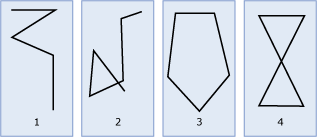# LineString

Applies to:SQL ServerAzure SQL DatabaseAzure SQL Managed Instance

A LineString is a one-dimensional object representing a sequence of points and the line segments connecting them.

## LineString Instances

The illustration below shows examples of LineString instances.As shown in the illustration:

• Figure 1 is a simple, nonclosed LineString instance.

• Figure 2 is a nonsimple, nonclosed LineString instance.

• Figure 3 is a closed, simple LineString instance, and therefore is a ring.

• Figure 4 is a closed, nonsimple LineString instance, and therefore is not a ring.

### Accepted Instances

Accepted LineString instances can be input into a geometry variable, but they may not be valid LineString instances. The following criteria must be met for a LineString instance to be accepted. The instance must be formed of at least two points or it must be empty. The following LineString instances are accepted.

``````DECLARE @g1 geometry = 'LINESTRING EMPTY';
DECLARE @g2 geometry = 'LINESTRING(1 1,2 3,4 8, -6 3)';
DECLARE @g3 geometry = 'LINESTRING(1 1, 1 1)';
``````

`@g3` shows that a LineString instance can be accepted, but not valid.

The following LineString instance is not accepted. It will throw a `System.FormatException`.

``````DECLARE @g geometry = 'LINESTRING(1 1)';
``````

### Valid Instances

For a LineString instance to be valid, it must meet the following criteria.

1. The LineString instance must be accepted.
2. If a LineString instance is not empty, then it must contain at least two distinct points.
3. The LineString instance cannot overlap itself over an interval of two or more consecutive points.

The following LineString instances are valid.

``````DECLARE @g1 geometry= 'LINESTRING EMPTY';
DECLARE @g2 geometry= 'LINESTRING(1 1, 3 3)';
DECLARE @g3 geometry= 'LINESTRING(1 1, 3 3, 2 4, 2 0)';
DECLARE @g4 geometry= 'LINESTRING(1 1, 3 3, 2 4, 2 0, 1 1)';
SELECT @g1.STIsValid(), @g2.STIsValid(), @g3.STIsValid(), @g4.STIsValid();
``````

The following LineString instances are not valid.

``````DECLARE @g1 geometry = 'LINESTRING(1 4, 3 4, 2 4, 2 0)';
DECLARE @g2 geometry = 'LINESTRING(1 1, 1 1)';
SELECT @g1.STIsValid(), @g2.STIsValid();
``````

Warning

The detection of LineString overlaps is based on floating-point calculations, which are not exact.

## Examples

### Example A.

The following example shows how to create a `geometry``LineString` instance with three points and an SRID of 0:

``````DECLARE @g geometry;
SET @g = geometry::STGeomFromText('LINESTRING(1 1, 2 4, 3 9)', 0);
``````

### Example B.

Each point in the `LineString` instance may contain Z (elevation) and M (measure) values. This example adds M values to the `LineString` instance created in the example above. M and Z can be null values.

``````DECLARE @g geometry;
SET @g = geometry::STGeomFromText('LINESTRING(1 1 NULL 0, 2 4 NULL 12.3, 3 9 NULL 24.5)', 0);
``````

### Example C.

The following example shows how to create a `geometry LineString` instance with two points that are the same. A call to `IsValid` indicates that the LineString instance is not valid and a call to `MakeValid` will convert the LineString instance into a Point.

``````DECLARE @g geometry
SET @g = geometry::STGeomFromText('LINESTRING(1 3, 1 3)',0);
IF @g.STIsValid() = 1
BEGIN
SELECT @g.ToString() + ' is a valid LineString.';
END
ELSE
BEGIN
SELECT @g.ToString() + ' is not a valid LineString.';
SET @g = @g.MakeValid();
SELECT @g.ToString() + ' is a valid Point.';
END
``````

Here is the result set.

``````LINESTRING(1 3, 1 3) is not a valid LineString
POINT(1 3) is a valid Point.
``````